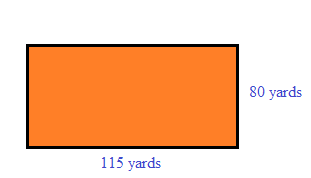### Home > MC1 > Chapter 2 > Lesson 2.2.4 > Problem2-96

2-96.

Three days each week Stacy exercises by walking around the soccer field near her home. The field is $80$ yards wide and $115$ yards long.

1. Draw a diagram of the field and find how far Stacy walks in one trip around the field (called the perimeter).

Your diagram might look something like this. Remember that the field is a rectangle, so sides opposite of each other are the same length.2. If Stacy walks around the field four times each time she exercises, how far does she walk each week?

Using the perimeter you found in part (a), you can easily find the distance Stacy walks each day. But remember, this question asks for how far Stacy walks each week.

Stacy walks $4680$ yards each week.

3. Additional Challenge: A mile is $1760$ yards. How many miles does Stacy walk each week?

To convert these units, it is best to use division. Find a way to write an expression using division to solve the problem.